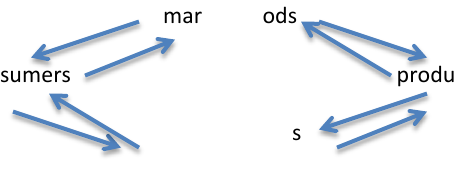# ECON 208 Chapter Notes - Chapter 2: Time Series, Panel Data, Scatter Plot

29 views3 pages
Page:
of 3Chapter 2 Economic Theory, Data, and Graphs
Sept.13.12
Positive and normative advice:
- Normative statements
- depend on value judgments and opinions
- use of facts
- subjective
- i.e. unemployment is a more important social problem than inflation
- Positive statements
- do not involve value judgments
- about what is, was, or will be
- objective
- can be tested
- i.e. the majority of the population would prefer a policy that reduced
unemployment to one that reduced inflation
- Disagreement among economists:
- failure to define their terms/points of reference clearly
- stems from economists’ failure to acknowledge the full state of their own
ignorance
- public disagreement based on the positive/normative distinction
- economists must differentiate normative advice from positive facts
- laws requiring equal pay for work of equal value will make women better off
- “better off” wages? Unemployment? Equality?
Theories:
- Constructed to explain things
- i.e. what determines quantity/price of eggs bought and sold this month
use theory of demand and supply
- theory consists of definitions about variables (endogenous, exogenous),
assumptions (motives, causation, applicability), and a set of
predictions/hypotheses
market goods
consumers producers
market factors
Models:
- Used as a synonym for a theory
- Applied to a specific quantitative formulation of a theory
- As an illustrative abstraction which helps to organize our thoughts about a
particular issue, not designed to generate testable hypotheses
Testing theories:
- Tested by confronting its predictions with evidence
- Scientific approach:
- empirical observation leads to construction of theories
- theories generate specific predictions
- the predictions are tested by more detailed empirical observation
- Rejection vs. confirmation
- prediction
- need confirming evidence
- an alternative is to see if the hypothesis can be rejected by data
Statistical analysis:
- Used to test a hypothesis (i.e. if x increases then y will also increase)
- Economists are compelled to use millions of “uncontrolled” experiments
going on every day
- Activities can be observed and recorded continuously, producing a mass of
data
- Analysis of this data requires the use of appropriate/complex statistical
techniques
Correlation vs. causation:
- Positive correlation means x and y move together
- Negative correlation means x and y move apart
- X and Y may not be causally related or they may be related in the opposite
way to what is expected (reverse causality)
- i.e. correlation: more ice cream trucks when the temperature is higher
causation: ice cream trucks don’t cause the temperature to be higher
- Need econometrics to prove causality
Economic data:
- Economists use data collected, i.e. governmental statistics
- Data can be displayed in tables and graphs
- When interested in relative movements we use index numbers
- Index numbers:
- used to compare changes in some variable relative to some base period
- important when the variables are measured in different units
- value of index in given period =
(absolute value in given period)/(absolute value in base period) x 100
- Economic variables come in two forms of data: cross-sectional and time-
series, the combination of the two is panel data
- A scatter diagram is a common way of looking at the relationship between
two variables
- Graphing economic theories:
- verbal statement
- numerical schedule (table)
- mathematical equation
- a graph
- Non-linear functions:
- recognize if the slope is positive or negative
- recognize if the absolute value of the slope is increasing or decreasing
- what happens to Y when X changes marginally
- what is the response of Y to marginal changes in X
- use absolute value and slope to answer these questions